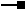ArcObjects Library Reference (Geometry)

# ITopologicalOperator3 Interface

Provides additional information on non-simple geometries. Note: the ITopologicalOperator3 interface has been superseded byITopologicalOperator5. Please consider using the more recent version.

#### Product Availability

Available with ArcGIS Engine, ArcGIS Desktop, and ArcGIS Server.

#### Members

DescriptionBoundary The boundary of this geometry. A polygon's boundary is a polyline. A polyline's boundary is a multipoint. A point or multipoint's boundary is an empty point or multipoint.Buffer Constructs a polygon that is the locus of points at a distance less than or equal to a specified distance from this geometry.Clip Constructs the intersection of this geometry and the specified envelope.ClipDense Constructs the intersection of this geometry and the specified envelope; densifies lines in output contributed by the clipping envelope.ClipToDomain Clips the geometry to the domain of the spatial reference. Useful for ensuring that buffers can be fit within the spatial domain of the feature class to which they are being added.ConstructUnion Defines this geometry to be the union of the inputs. More efficient for unioning multiple geometries than calling Union repeatedly.ConvexHull Constructs the convex hull of this geometry.Cut Splits this geometry into a part left of the cutting polyline, and a part right of it.Difference Constructs the geometry containing points from this geometry but not the other geometry.GeoNormalizeEx Shifts longitudes, if need be, into a continuous range of 360 degrees.Intersect Constructs the geometry that is the set-theoretic intersection of the input geometries. Use different resultDimension values to generate results of different dimensions.IntersectMultidimension Constructs the set-theoretic intersection of the inputs. The results are returned in a geometry bag with one element per result dimension.IsKnownSimple Indicates whether this geometry is known (or assumed) to be topologically correct.IsKnownSimple Indicates whether this geometry is known (or assumed) to be topologically correct.IsSimple Indicates whether this geometry is known (or assumed) to be topologically correct, after explicitly determining this if the geometry is not already known (or assumed) to be simple.IsSimpleEx Determines why a geometry is not simple. Currently only implemented for polygons.QueryClipped Redefines clippedGeometry to be the intersection of this geometry and the clipping envelope.QueryClippedDense Redefines clippedGeometry to be the intersection of this geometry and the clipping envelope; densifies lines in the output contributed by the clipping envelope.Simplify Makes this geometry topologically correct.SymmetricDifference Constructs the geometry that contains points from either but not both input geometries.Union Constructs the geometry that is the set-theoretic union of the input geometries.

#### Inherited Interfaces

Interfaces Description
ITopologicalOperator2 Provides access to members that extend the ITopologicalOperator interface.
ITopologicalOperator Provides access to members for constructing new geometries based upon topological relationships between existing geometries.

#### CoClasses that implement ITopologicalOperator3

CoClasses and Classes Description
MultiPatch A collection of surface patches.
Polygon A collection of rings ordered by their containment relationship; optionally has measure, height and ID attributes.
Polyline An ordered collection of paths; optionally has measure, height and ID attributes.

#### Remarks

ITopologicalOperator3 methods must be applied on high-level geometries only. High-Level geometries are point, multipoint, polyline and polygon. To use this interface with low-level geometries such as segments (Line, Circular Arc, Elliptic Arc, Bezier Curve), paths or rings, they must be wrapped into high-level geometry types.